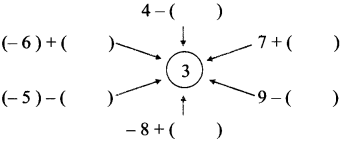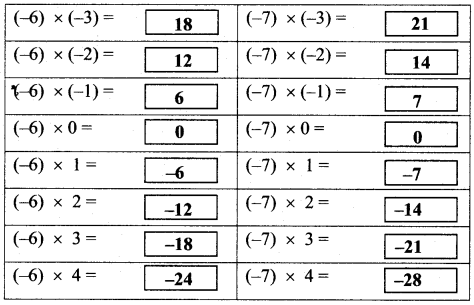# Maharashtra Board Class 7 Maths Solutions Chapter 2 Multiplication and Division of Integers Practice Set 8

## Maharashtra State Board Class 7 Maths Solutions Chapter 2 Multiplication and Division of Integers Practice Set 8

Question 1.
Multiply:

1. (-5) × (-7)
2. (-9) × (6)
3. (9) × (-4)
4. (8) × (-7)
5. (-124) × (-1)
6. (-12) × (-7)
7. (-63) × (-7)
8. (-7) × (15)

Solution:

1. 35
2. -54
3. -36
4. -56
5. 124
6. 84
7. 441
8. -105

Maharashtra Board Class 7 Maths Chapter 2 Multiplication and Division of Integers Practice Set 8 Intext Questions and Activities

Question 1.
In the previous class, we have learnt to add and subtract integers. Using those methods, fill in the blanks below. (Textbook pg. no. 11)

1. 5 + 7 = __
2. 10 + (-5) = __
3. -4 + 3 = __
4. (-7) + (-2) = __
5. (+8) – (+ 3) = __
6. (+8) – (-3) = __

Solution:

1. 12
2. 5
3. -1
4. -9
5. 5
6. 11

Question 2.
Write a number in each bracket to obtain the answer ‘3’ in each operation. (Textbook pg. no. 11)Solution:Question 3.
Multiply the given integers and complete the table given below. (Textbook pg. no. 12)Solution: